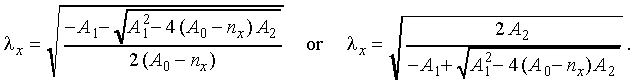# The calibration of the monochromator UM-2

Hans Korge

In the manuals of the practical works [Optika praktikum VII. Koostanud A.Haav. Tartu, TÜ, 1990, lk.58-67 or Praktikum po optike VII. Sostavitel` A.Haav. Tartu, TU, 1991, s.63-73] the calibration of the monochromator is based on the construction of the monochromator drum reading versus wavelength plot on a diagram paper with the following determination of the unknown wavelength of spectral lines from this plot. The calibration and the determination can be done more readily and with greater accuracy using a PC.

As we know, in the position of the minimum deviation the ray passes through the prism symmetrically (Figure 1):Figure 1Consequently, in this position the angle of refractionis always, whereis the prism refracting angle (the apex angle), and due to the prism material dispersionthe angle of incidencemust be different for different wavelengths:In the monochromator UM-2 the Abbe prism is used, that means that the refracting flint-glass prism is cut into halves along the apex angle bisector (prisms 1' and 1'') and these parts are glued together with a total reflection prism 2 in the way shown in Figure 2.Figure 2

Such an arrangement ensures that in the minimum of deviation the incident and deviated rays are perpendicular to each other. Therefore to find the right angle of incidence at a given wavelength, the prism is rotated around his axis with the help of micrometer screw (a big cylindrical drum provided with the scale).

For the calibration one must determine the correspondence between the drum scale reading N and the wavelength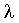, but the respective relation is not linear. Linear is the relation between the scale reading, which determines the prism position with respect to incident beam, and the corresponding angle of incidence. Hence it follows an idea for the monochromator calibration and the determination of the wavelengths of unknown spectral lines by the computation.

The refracting prism of the monochromator UM-2 is made of the heavy flint glass TF3 which standard dispersions are as follows [A.S.Toporets. Monokhromatory. M.,1955]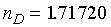and the Abbe number.

The standard indices of refraction of the glass TF3 can also be used [K.I.Tarasov. Spektral`nye pribory. L., 1977]:

Table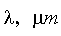nn 0.3650 1.786 12 0.5461 1.723 17 0.4046 1.762 14 0.6563 1.710 37 0.4341 1.749 89 0.7665 1.702 88 0.4861 1.734 68 0.8630 1.698 48

Using different initial data we get somewhat different results, but also the possibility to try out which data match in the best way with our measurements. The spectral lines with the known wavelengths are suitable for decision, and they are used for calibration.

The computation is made up of the following steps:

1) Having calculated the indices of refraction corresponding to the Fraunhofer lines C, D, F and G, or using the data from the table we can draw up a system of quadratic equations relying on the Cauchy formula:(Using the data from the table one must content with the minimal necessary interval of wavelengths because the greater the interval is, the less accurate is the polynomial approximation.)
Denoting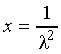and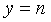, this system looks like as follows: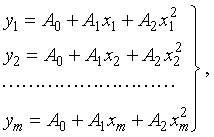and resolving it by the method of least squares, we get the best fitting coefficients A0, A1, A2 of the Cauchy formula: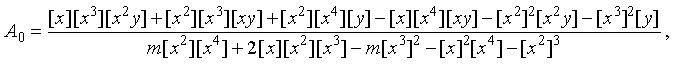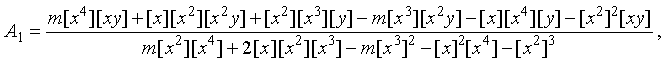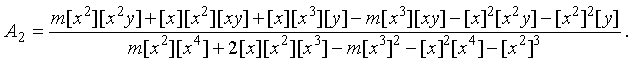Here the Gauss brackets enable to shorten the writing of formulas: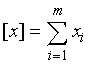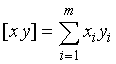, etc.

2) Knowing the coefficients of the Cauchy formula one can calculate at first the refractive indexof the prism material for the given wavelengthand then the corresponding angle of incidenceat the minimum of deviation because the refracting angle of prism is also known ().

3) Using the mercury or the mercury-cadmium spectral lamp and recording the scale readings, corresponding to all spectral lines, one can compute the coefficients of regression formula relying on the easily recognizable Hg-lines: 690.7, 623.4, 579.1, 577.0, 491.6, 435.8, 404.7 and those of Cd-lines: 643.8, 508.6, 480.0, 467.8 nm. To obtain the regression coefficients we must at first calculate the refractive indices ni corresponding to the recognized Hg-lines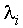, and then the angles of incidenceThe regression coefficients a and b are calculated from the system of equations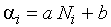,

where Ni is the drum scale reading, corresponding to the wavelength.

4) The determination of the unknown line wavelengths proceeds in the reverse order. For the scale reading Nx we find the corresponding angle of incidence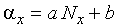,

then the refractive index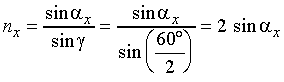,

and finally the wavelength to be sought from the Cauchy formula